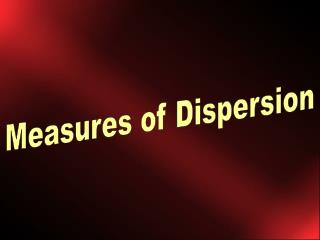Download PresentationMeasures of Dispersion

# Measures of Dispersion - PowerPoint PPT PresentationDownload Presentation## Measures of Dispersion

- - - - - - - - - - - - - - - - - - - - - - - - - - - E N D - - - - - - - - - - - - - - - - - - - - - - - - - - -
##### Presentation Transcript

1. Measures of Dispersion

2. Learning Objectives • In this chapter you will learn • about the importance of variation • how to measure variation • range • variance • standard deviation

3. Variation • Variation • is the heart of statistics • no variation, no need to do statistical analysis • the mean would describe the distribution

4. Quest for Variation • Measures of dispersion • consider the spread between scores • Calculations include • range • variance • standard deviation

5. The Range • Range • distance between the highest and lowest score in a distribution. • Calculation • Range = H minus L • H = the highest score in the data set • L = the lowest score in the data set

6. Variance and Standard Deviation • Most commonly used measures of dispersion • based upon the distance of scores in a distribution from the mean • the mean is used as the central point • first step is calculation of deviation scores • how each score stands in relation to the mean

7. Calculation • Formula: x = (X minus the Mean) • Table 4.2 reveals how this process works • we have the number of prior drug arrests for five clients who appeared in Tuesday’s drug court

8. X f fX x ( x – X) 6 1 6 2 5 1 5 1 4 1 4 0 3 1 3 - 1 2 1 2 - 2 N = 5 ∑fX = 20 ∑x = 0 Table 4.2: Number of Prior Arrests for Drug Offenses Among Tuesday’s Drug Court Clients

9. Calculation • The mean number of prior drug arrests was four • next step is to calculate how far each score (person in this case) stood in relation to this mean of four. • The deviation score (x) is calculated by subtracting the mean from each score in the distribution

10. Calculation • Next step • on the first line of Table 4.2 is the score 6. If we subtract the mean, the deviation score is 2 • this person had two more prior drug arrests than the average person appearing before the drug court on Tuesday • repeat this process from each score in the distribution

11. Check the Math • One characteristics of the mean is that the sum of the deviations from it equals zero (x = 0) • The sum of the deviations from the mean equal zero

12. Characteristic • Positive deviation scores of • the values above the mean • are cancelled out • by the negative deviation scores of the values that fall below the mean • We are left with zero • when we sum up the deviation scores in a distribution • except for rounding or calculation errors

13. Squaring the Distance • In order to remove the negative signs • square every deviation score and thus cancel out the negative numbers • remember that a negative value times a negative value equals a positive value • squaring the deviation scores gives us a total number we can work with – the variance

14. The Variance • A new frequency distribution • based upon how each case stands in relation to the mean • We can calculate another average score – the variance • The variance is the mean of the squared deviations from the mean

15. Variance • The previous definition is • a verbal formula • a description of how the variance is calculated • The variance is a mean • it represents the average squared deviations • that each score stands in relation to its mean

16. Variance • The variance is • a measure of the spread of scores in a distribution around its mean • The larger the variance • the greater the spread of scores around the mean • The smaller the variance • the more closely the scores are distributed around the mean

17. Standard Deviation • We squared the deviation scores around the mean in order to clear the negative numbers • The standard deviation is the square root of the variance

18. Standard Deviation • Standard deviation • is a measure of dispersion of the scores around the mean • The higher the standard deviation • the greater the spread in the scores • The lower the standard deviation • the closer the scores are on average from the mean of the distribution

19. Formulae Population variance Population standard deviation Sample variance Sample standard deviation

20. Summary • Explaining variation is the basis for statistical analysis • We begin with basic measures of dispersion • range • variance • standard deviation

21. SPSS Open the SPSS data file Select ANALYZE Select DESCRIPTIVE STATISTICS Select FREQUENCIES

22. SPSS Select the variable from the column on the right Highlight it Mouse click the ARROW button to move it in the VARIABLES window

23. SPSS Select the appropriate measures of central tendency and, shape and dispersion Mouse click CONTINUE